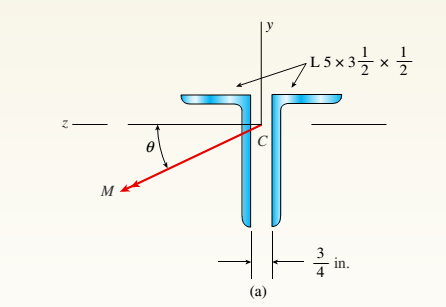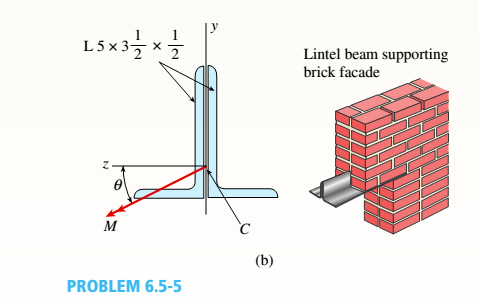# A beam made up all woun equal leg angles is subjected to a bending moment M having its vector .u an angle (i) lo lire axis (see figure paria). (a) For the position shown in lire figure, determine lire orienlalion of lire neulral axis and calculate lire maximum tensile s'av-s ir, and maximum compressive stress (b) The two angles are now inverted and attached back-lo-back lo lorn, a lintel beam that supports two courses of brick facade i see figure part b). Find the new orientation of the neutral axis and calculate the maximum tensile slress r. a::d maximum compressive s'avsrr . in I he beam using 6 = 30° and M = 30 kip-in.### Mechanics of Materials (MindTap Co...

9th Edition
Barry J. Goodno + 1 other
Publisher: Cengage Learning
ISBN: 9781337093347

#### Solutions

Chapter
Section### Mechanics of Materials (MindTap Co...

9th Edition
Barry J. Goodno + 1 other
Publisher: Cengage Learning
ISBN: 9781337093347
Chapter 6, Problem 6.5.5P
Textbook Problem
120 views

## A beam made up all woun equal leg angles is subjected to a bending moment M having its vector .u an angle (i) lo lire axis (see figure paria).(a) For the position shown in lire figure, determine lire orienlalion of lire neulral axis and calculate lire maximum tensile s'av-s ir, and maximum compressive stress (b) The two angles are now inverted and attached back-lo-back lo lorn, a lintel beam that supports two courses of brick facade i see figure part b). Find the new orientation of the neutral axis and calculate the maximum tensile slress r. a::d maximum compressive s'avsrr . in I he beam using 6 = 30° and M = 30 kip-in.(a)

To determine

Orientation of neutral axis and maximum tensile and compressive stress.

### Explanation of Solution

Given Information:

The following figure is given,

Also, θ=30 and M=30 kip-in.. is given.

The data of L 5×312×12 beam

are as,

Area=4 in.2,width =312 in.,  depth=5 in.c=0.901 in., d=1.65 in.,I1=10 in.4, I2=4.02 in.4.

Neutral axis lies on the zero normal stress line.

The normal stress is given by,

σx=MyzIyMzyIz

Equate it to zero to find the line.

Calculation:

Consider the following figure,

Axis 1 is z -axis and axis 2 is y -axis.

Moments of area of the whole cross section are,

Iz=2I1=20 in4Iy=2[I2+A( c+ 3 8)2]=2[4.02+4( 0.9+ 3 8)2]=21

(b)

To determine

Orientation of neutral axis and maximum tensile and compressive stress.

### Still sussing out bartleby?

Check out a sample textbook solution.

See a sample solution

#### The Solution to Your Study Problems

Bartleby provides explanations to thousands of textbook problems written by our experts, many with advanced degrees!

Get Started

Find more solutions based on key concepts
What type of toolholder might he selected for mounting a slitting saw?

Precision Machining Technology (MindTap Course List)

What are the basic characteristics of a NoSQL database?

Database Systems: Design, Implementation, & Management

Explain why database design is important.

Database Systems: Design, Implementation, & Management

Give examples of the role of motors in our daily life.

Engineering Fundamentals: An Introduction to Engineering (MindTap Course List)

What is a system requirement, and how are system requirements classified?

Systems Analysis and Design (Shelly Cashman Series) (MindTap Course List)

Differentiate between a PC and a mobile computer. A laptop also is known as a(n) ___ computer.

Enhanced Discovering Computers 2017 (Shelly Cashman Series) (MindTap Course List)

What determines the exact temperature of the shielded metal welding arc?

Welding: Principles and Applications (MindTap Course List)

If your motherboard supports ECC DDR3 memory, can you substitute non-ECC DDR3 memory?

A+ Guide to Hardware (Standalone Book) (MindTap Course List)